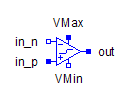Multiphase Ideal Intermediate Switch - MapleSim Help

Multiphase Ideal Intermediate Switch

Multiphase ideal intermediate switchDescription The Multiphase Ideal Intermediate Switch (or Ideal Intermediate Switch) component contain $m$ single-phase ideal intermediate switch models, each connected between corresponding phases of ${\mathrm{plug}}_{\mathrm{p1}}$, ${\mathrm{plug}}_{\mathrm{p2}}$, ${\mathrm{plug}}_{\mathrm{n1}}$, and ${\mathrm{plug}}_{\mathrm{n2}}$, with the control from the corresponding signal in the $\mathrm{control}$ vector input. A vector of optional thermal ports, one for each switch, is enabled if Use Heat Port is true.Connections

 Name Description Modelica ID ${\mathrm{plug}}_{\mathrm{p1}}$ Port 1 positive $m$-phase plug plug_p1 ${\mathrm{plug}}_{\mathrm{p2}}$ Port 2 positive $m$-phase plug plug_p2 ${\mathrm{plug}}_{\mathrm{n2}}$ Port 1 negative $m$-phase plug plug_n2 ${\mathrm{plug}}_{\mathrm{n1}}$ Port 2 negative $m$-phase plug plug_n1 $\mathrm{control}$ Boolean input vector control $\mathrm{Heat Port}$ heatPortParameters

 Name Default Units Description Modelica ID $m$ $3$ Number of phases m ${R}_{\mathrm{on}}$ * $1·{10}^{-5}$ $\mathrm{\Omega }$ Closed switch resistance Ron ${G}_{\mathrm{off}}$ * $1·{10}^{-5}$ $S$ Opened switch conductance Goff Use Heat Port $\mathrm{false}$ True (checked) means heat ports are enabled useHeatPort

* Starred values are vector parameters; the default is $\mathrm{fill}\left(x,m\right)$, which creates a vector with $m$ entries of $x$.Modelica Standard Library The component described in this topic is from the Modelica Standard Library. To view the original documentation, which includes author and copyright information, click here.